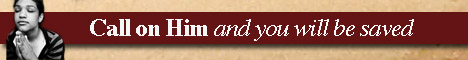Note:  Do not rely on this information. It is very old.

# Quadrature

Quadrature really means the determination of a square whose area shall be equal to that of any given surface. The area of such surface can, however, be found without the actual determination of the equivalent square; hence the term quadrature is now applied to any process which gives a means of expressing the area in terms of any definite unit. The quadrature, or squaring of the circle, is a problem which has excited much attention from most remote times, and is often classed with that of finding perpetual motion. Since the area of the circle could be expressed in terms of the radius and circumference, the problem really became to find geometrically a straight line equal to the circumference, or to find the ratio pi between circumference and diameter. Many people, ignorant of the nature of the problem, have believed that they have "squared the circle" in some simple way; but Lambert and others proved - what was long suspected - that the number pi was incommensurable. One geometrical method of approximation is to inscribe a square in a circle and to add one-fifth of the side of the square to three times the diameter of the circle. A curious method adopted by Mr. A. Smith and others was to toss a thin rod many times on a level floor on which a number of parallel lines were drawn at equal distances. If the length of the rod be c and the distance between the lines be d, the probability that the rod will lie across a line is 2 c/pi d. By actually counting the number of times that this result, occurred in 3,200 tosses the value 3.1553 was obtained for pi. For a general discussion of the subject the reader is referred to Mathematical Recreations and Problems by W. W. R. Bell.